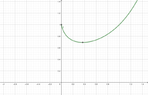# Calculate (and argue) the critical points of an exponential function

Gold Member
Homework Statement:
Calculate the critical point of ##y=x^x##
Relevant Equations:
Chain Rule, Product Rule
Hi everybody

We can't differentiate ##x^x## neither like a power function nor an exponential function. But ##x^x=e^{x\mbox{ln}x}##. So

##\dfrac{d}{dx}x^x=\dfrac{d}{dx}e^{x\mbox{ln}x}=x^x(\mbox{ln}x+1)##

And here comes the doubt: prove the domain of ##x^x## is ##(0, +\infty)##

Why is only positive?; why it will never be 0?

So ##(\mbox{ln}x+1)=0\Rightarrow{x=1/e}##

It's a solved exercise at Calculus, by R.A.A. It's the only question not solved (the domain, I mean).

Homework Helper
Gold Member
2022 Award
You can define ##0^0## if you want, but fractional powers of negative numbers are undefined, or require complex numbers. E.g. ##-0.5^{-0.5}## is not a real number.

•mcastillo356
Gold Member
Can ##0^0=1##? The book says: ##x^x## is never 0. This is the graph:##0^x=0## on one hand; ##x^0=1##. This is all I can provide.Mentor
2022 Award
##0^0## is given a value per definition. If you want continuity of ##x \longrightarrow x^0## at ##x=0## then you have to set ##0^0=1,## and if you want continuity of ##x\longrightarrow 0^x## at ##x=0## then you have to set ##0^0=0.##

My personal opinion is, that only ##0^0=1## is a valid definition because exponentiation is an abbreviation for multiplication and all multiplications over an empty set of indexes equals the neutral element of multiplication, which is ##1.##
$$\sum_{i\in \{\}} a_i = 0 \quad ;\quad \prod_{i\in \{\}} a_i = 1$$

Last edited:
•mcastillo356
Gold Member
The next step would be to prove ##\displaystyle\lim_{x \to{0+}}{x^x}=f(0)=1##? I am just wondering.

Mentor
2022 Award
The next step would be to prove ##\displaystyle\lim_{x \to{0+}}{x^x}=f(0)=1##? I am just wondering.
Yes. E.g. we get for ##x>0##
$$\lim_{n \to \infty}\left(\dfrac{1}{n}\right)^{1/n}= \lim_{n \to \infty}\left(1-\dfrac{\log n}{n}+O(n^{-2})\right)=1.$$
But if you decouple base and exponent, say ##f(x,y)=x^y,## then all depends on which path you approach ##(0,0).## That is where the question: "Why isn't ##0^0=0## ?" comes from. ##x\longmapsto x^x ## and ##(x,y)\longmapsto x^y## are different functions with different properties. And even ##f(x,y)## depends on its domain: real positive numbers, all real numbers and complex values, complex numbers and complex values. These are questions that should be answered before we discuss ##0^0.## The answer depends on them.

•mcastillo356
Gold Member
##\displaystyle\lim_{x \to{0^+}}{e^{x\ln(x)}}=e^{\lim_{x \to{0^+}}{x\ln(x)}}=e^{\lim_{x \to{0^+}}{\frac{\ln(x)}{1/x}}}##, and, by L'Hopital, ##\displaystyle e^{\lim_{x \to{0^+}}{-x}}=1##.

Is this equal to ##f(0)##? Don't know. ##x^x=e^{x\ln(x)}##. Does this alow me to say "no", because ##\ln 0## is nonsense?.

Mentor
2022 Award
##\displaystyle\lim_{x \to{0^+}}{e^{x\ln(x)}}=e^{\lim_{x \to{0^+}}{x\ln(x)}}=e^{\lim_{x \to{0^+}}{\frac{\ln(x)}{1/x}}}##, and, by L'Hopital, ##\displaystyle e^{\lim_{x \to{0^+}}{-x}}=1##.
This is correct.
Is this equal to ##f(0)##? Don't know. ##x^x=e^{x\ln(x)}##. Does this alow me to say "no", because ##\ln 0## is nonsense?.
What do you mean? "No" as an answer to what? And where do you have ##\log 0## if you use de L'Hôpital?
You could as well use a Taylor expansion of ##\log## and observe that ##y=x## goes faster to zero than ##y=\log x ## does so that the product goes to zero. Taylor will give you a quantitative measure for "faster than".

Last edited:
•mcastillo356
Gold Member
Another tryIn the exercise I'm given a function ##f(x)=x^x##. We have ##x^x:=e^{x\ln x}##, which is well defined for ##x>0##, so the domain is ##(0,\infty)##.

Now, we can define another function ##[0,\infty)\rightarrow{\mathbb R}## such that

$$g(x):=\begin{cases}{f(x)}&\text{if}& x\in{(0,\infty)}\\1 & \text{if}& x=0\end{cases}$$

Where we assume ##0^0:=1##

Mentor
2022 Award
You can define ##g(x)## whichever you want. The question is: which properties do you require? If you do it like this, then we get a continuous extension of ##f(x)##.

•mcastillo356
Gold Member
Hi, fresh_42

The question is: which properties do you require?
No idea. I'm afraid I let myself struggle with maths with lots of naivety. Sooo...PF is a great, great, help, and a place where I feel at home, an island of give-and-take knowledge.

But I think I generate expectations I'm not able to tackle...

Conclusion: Thanks for helping me one more time. Much love. Forgive me if this post is improper. I felt I had to contextualize the thread.

•berkeman and fresh_42